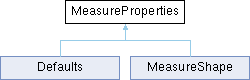LibreOffice LibreOffice 7.4 SDK API Reference
MeasureProperties Service Referencepublished

This service describes a MeasureShape. More...

`import"MeasureProperties.idl";`

Inheritance diagram for MeasureProperties:## Public Attributes

boolean MeasureBelowReferenceEdge
If this property is `TRUE`, the measure is drawn below the reference edge instead of above it. More...

long MeasureHelpLine1Length
This is the length of the first help line. More...

long MeasureHelpLine2Length
This is the length of the second help line. More...

long MeasureHelpLineDistance
This is the distance from the measure line to the start of the help lines. More...

long MeasureHelpLineOverhang
This is the overhang of the two help lines. More...

com::sun::star::drawing::MeasureKind MeasureKind
This enumeration specifies the MeasureKind. More...

long MeasureLineDistance
This is the distance from the reference edge to the measure line. More...

long MeasureOverhang
This is the overhang of the reference line over the help lines. More...

boolean MeasureShowUnit
If this is `TRUE`, the unit of measure is shown in the measure text. More...

boolean MeasureTextAutoAngle
If this is `TRUE`, the angle of the measure is set automatically. More...

long MeasureTextAutoAngleView
This is the automatic angle. More...

long MeasureTextFixedAngle
This is the fixed angle. More...

com::sun::star::drawing::MeasureTextHorzPos MeasureTextHorizontalPosition
This is the horizontal position of the measure text. More...

com::sun::star::drawing::MeasureTextVertPos MeasureTextVerticalPosition
This is the vertical position of the text. More...

boolean MeasureTextIsFixedAngle
If this value is `TRUE`, the measure has a fixed angle. More...

boolean MeasureTextRotate90
If this value is `TRUE`, the text is rotated 90 degrees. More...

boolean MeasureTextUpsideDown
If this value is `TRUE`, the text is printed upside down. More...

short MeasureDecimalPlaces
This value is the number of decimal places that is used to format the measure value. More...

## Detailed Description

This service describes a MeasureShape.

## ◆ MeasureBelowReferenceEdge

 boolean MeasureBelowReferenceEdge
property

If this property is `TRUE`, the measure is drawn below the reference edge instead of above it.

## ◆ MeasureDecimalPlaces

 short MeasureDecimalPlaces
optionalproperty

This value is the number of decimal places that is used to format the measure value.

Since
OOo 1.1.2

## ◆ MeasureHelpLine1Length

 long MeasureHelpLine1Length
property

This is the length of the first help line.

## ◆ MeasureHelpLine2Length

 long MeasureHelpLine2Length
property

This is the length of the second help line.

## ◆ MeasureHelpLineDistance

 long MeasureHelpLineDistance
property

This is the distance from the measure line to the start of the help lines.

## ◆ MeasureHelpLineOverhang

 long MeasureHelpLineOverhang
property

This is the overhang of the two help lines.

## ◆ MeasureKind

 property

This enumeration specifies the MeasureKind.

## ◆ MeasureLineDistance

 long MeasureLineDistance
property

This is the distance from the reference edge to the measure line.

## ◆ MeasureOverhang

 long MeasureOverhang
property

This is the overhang of the reference line over the help lines.

## ◆ MeasureShowUnit

 boolean MeasureShowUnit
property

If this is `TRUE`, the unit of measure is shown in the measure text.

## ◆ MeasureTextAutoAngle

 boolean MeasureTextAutoAngle
property

If this is `TRUE`, the angle of the measure is set automatically.

## ◆ MeasureTextAutoAngleView

 long MeasureTextAutoAngleView
property

This is the automatic angle.

## ◆ MeasureTextFixedAngle

 long MeasureTextFixedAngle
property

This is the fixed angle.

## ◆ MeasureTextHorizontalPosition

 com::sun::star::drawing::MeasureTextHorzPos MeasureTextHorizontalPosition
property

This is the horizontal position of the measure text.

## ◆ MeasureTextIsFixedAngle

 boolean MeasureTextIsFixedAngle
property

If this value is `TRUE`, the measure has a fixed angle.

## ◆ MeasureTextRotate90

 boolean MeasureTextRotate90
property

If this value is `TRUE`, the text is rotated 90 degrees.

## ◆ MeasureTextUpsideDown

 boolean MeasureTextUpsideDown
property

If this value is `TRUE`, the text is printed upside down.

## ◆ MeasureTextVerticalPosition

 com::sun::star::drawing::MeasureTextVertPos MeasureTextVerticalPosition
property

This is the vertical position of the text.

The documentation for this service was generated from the following file: